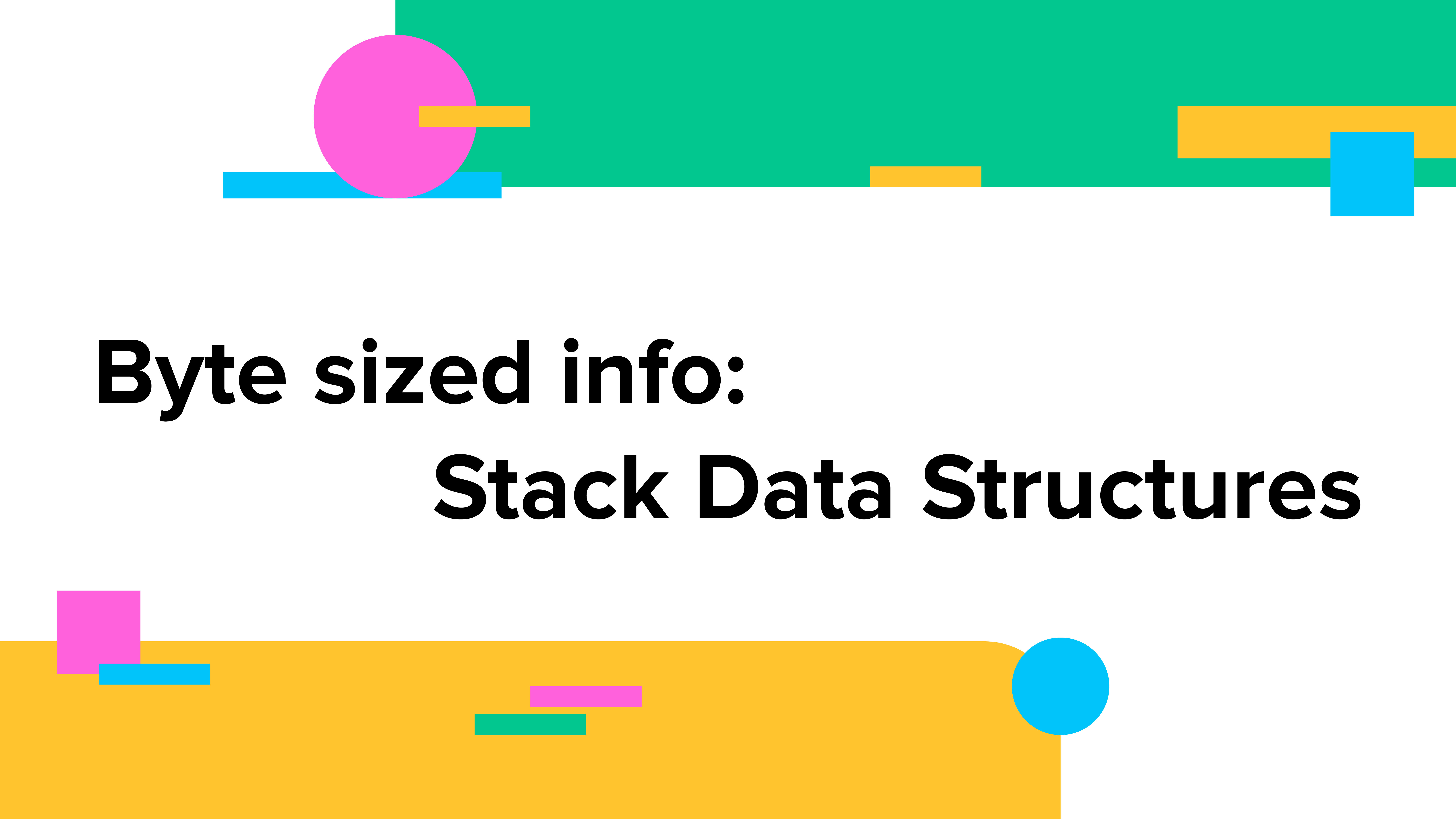# Byte Size Info: Stack Data StructuresOne of the first linear structures that we will be talking about is stacks. To re-iterate:

In a linear data structure all the elements are sequentially arranged one after the other. Therefore each element is connected to elements before and after. These are organized as a single level and users can traverse through the elements of a linear data structure in a single run. Linear data structures are mainly used in software applications.

### Characteristics of Stack Data Structure

Stacks are an ordered list of elements. They follow the last in, first out (LIFO) principle. This means that the last element to be added to a stack is the first element that will be removed from it. Much like a stack of pancakes, the last pancake to be placed on the plate is the first to be eaten.  A stack can have any abstract data type as an element contained within it which is why you will also notice it being categorized as an abstract data type.  Taking short detour, an abstract data type is one whose implementation is hidden from us. Stacks have two principle operations, called push  and pop which refer to adding and removing elements from a stack. These two operations happen at the same end of a stack. So the first element to be added to a stack will be the last one to be removed. Similarly, the last item to be added to the stack will be the first one to be removed.

#### Where are stacks used

Stacks are used to to process function calls, store variables in a computer’s memory, evaluate math expressions and more.

#### Stack operations

Keeping in mind the LIFO principle, the following are operations that can be done on a stack data structure. You may be acquainted with some of these operations such as push(), pop() etc:

• insertion/push(): adding elements to a stack. Adding and removing elements to a stack happens at the same location which is the top of the stack.
• deletion/pop(): removing elements from a stack. This happens at the same location as adding an element  which is the top of the stack.
• peek(): taking a peek at the top most element in a stack without removing it
• isEmpty(): true will be returned is the stack is empty. Else false is returned
• clear(): removing all elements from a stack
• size(): returns the number of items in a stack
• display(): display all the elements in a stack
• change(): change an element at a given position in a stack

#### Implementation of stack data structure

A stack data structure can be implemented using a one-dimensional array and linked list.

#### Arrays

Let’s use some common stack operations on an array using JavaScript, starting with the insertion operation using the push() which adds an element to the end of an array:

``````let array1 = [1,2,3,4];
array1.push(5);
//[1,2,3,4,5``````

Similarly we can use the pop() method for the deletion operation which will remove/pop off an element from the end of an array:

``````array1.pop();
//[1,2,3,4]``````

The peek operation lets us access the top post element of a stack:

``````let array1 = [1,2,3,4];
console.log(array1.length -1) //3``````

We can clear all the elements in a stack by resetting the the array:

``let array1 = []``

We can also iterate through the array and use the pop() method to keep removing the last element of an array for the length of the array. The length of an array refers to the number of items in an array. For example:

``````let array1 = [1,2,3,4];
for(let i = 0; i = array1.length; i++){
array1.pop()
}
console.log(array1);// []``````

There is no native isEmpty() method in JavaScript to check whether an array is empty, instead we can check if the length property of the array is greater than 1:

``array1.length === 0; //array is empty``

To  change the element at a particular position in an array, we will reference the particular array index and then change the value at said index:

``array1 = "a"; //["a",2,3,4] ``

With all the above information, now let’s construct a stack class in JavaScript in which we will implement all the above methods from scratch:

``````class Stack {

constructor() {
this.items = [];
}
//JS has a built in push() so let's use that
push(el) {
this.items.push(el)
}
//JS has a built in pop() so let's use that
pop(el) {
if (this.el.length === 0) {
return false;
} else {
this.el.pop()
}
}
//peek
peek() {
return this.items[this.items.length - 1];
}

//clear

clear() {
this.items = []
}
//display all elements of the stack to the console
display() {
for (var i = 0; i < this.items.length; i++) {
console.log(this.items[i])
}
}
//size(): get the size of an array by querying the length property
size() {
console.log(this.items.length);
}

//empty(): return true if the length of the array is 0
empty() {
if (this.items.length < 1) {
console.log("The array is empty");
} else {
return true
}
}
}``````